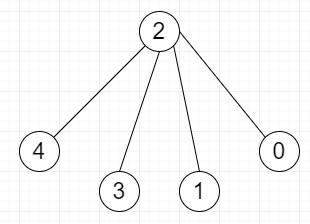# Find if an undirected graph contains an independent set of a given size in Python

Suppose we have a given undirected graph; we have to check whether it contains an independent set of size l. If there is any independent set of size l then return Yes otherwise No.

We have to keep in mind that an independent set in a graph is defined as a set of vertices which are not directly connected to each other.

So, if the input is like L = 4,then the output will be yes

To solve this, we will follow these steps −

• Define a function is_valid() . This will take graph, arr

• for i in range 0 to size of arr, do

• for j in range i + 1 to size of arr, do

• if graph[arr[i], arr[j]] is same as 1, then

• return False

• return True

• Define a function solve() . This will take graph, arr, k, index, sol

• if k is same as 0, then

• if is_valid(graph, arr) is same as True, then

• sol := True

• return

• otherwise,

• if index >= k, then

• return(solve(graph, arr[from index 0 to end] concatenate a list with [index], k-1, index-1, sol) or solve(graph, arr[from index 0 to end], k, index-1, sol))

• otherwise,

• return solve(graph, arr[from index 0 to end] concatenate a list with [index], k-1, index-1, sol)

## Example

Let us see the following implementation to get better understanding −

Live Demo

def is_valid(graph, arr):
for i in range(len(arr)):
for j in range(i + 1, len(arr)):
if graph[arr[i]][arr[j]] == 1:
return False
return True
def solve(graph, arr, k, index, sol):
if k == 0:
if is_valid(graph, arr) == True:
sol = True
return
else:
if index >= k:
return (solve(graph, arr[:] + [index], k-1, index-1, sol) or solve(graph, arr[:], k, index-1, sol))
else:
return solve(graph, arr[:] + [index], k-1, index-1, sol)

graph = [
[1, 1, 0, 0, 0],
[1, 1, 1, 1, 1],
[0, 1, 1, 0, 0],
[0, 1, 0, 1, 0],
[0, 1, 0, 0, 1]]
k = 4
arr = []
sol = [False]
solve(graph, arr[:], k, len(graph)-1, sol)
if sol:
print("Yes")
else:
print("No")

## Input

[[1, 1, 0, 0, 0],
[1, 1, 1, 1, 1],
[0, 1, 1, 0, 0],
[0, 1, 0, 1, 0],
[0, 1, 0, 0, 1]],
4

## Output

Yes